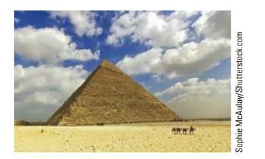Chapter 1, Problem 30P

Chapter
Section
Textbook Problem

The base of a pyramid covers an area of 13.0 acres (1 acre = 43 560 ft2) and has a height of 481 ft (Fig. P1.30). If the volume of a pyramid is given by the expression V = bh/3, where b is the area of the base and h is the height, find the volume of this pyramid in cubic meters.Figure P1.30

To determine
The volume of the pyramid.

Explanation

Given Info: The base of the pyramid is 13acres and the height o f the pyramid is 481ft .

Explanation:

Formula to calculate the volume of the pyramid is,

V=13bh

• V is volume of the pyramid
• b is base of the pyramid,
• h is the height of the pyramid

From unit transformation,

1acre=43560ft2

1ft3=2.832×102m3

Substitute 13acres for b , 481ft for h to find V

Still sussing out bartleby?

Check out a sample textbook solution.

See a sample solution

The Solution to Your Study Problems

Bartleby provides explanations to thousands of textbook problems written by our experts, many with advanced degrees!

Get Started

Both carbohydrates and protein have 4 calories per gram. T F

Nutrition: Concepts and Controversies - Standalone book (MindTap Course List)

How do a solute, a solvent, and a solution differ?

Biology: The Dynamic Science (MindTap Course List)

How can errors in the cell cycle lead to cancer in humans?

Human Heredity: Principles and Issues (MindTap Course List)